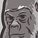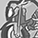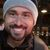# QlikView App Dev

Discussion Board for collaboration related to QlikView App Development.

Announcements
QlikWorld happening right now! You can still join: REGISTER NOW
cancel
Showing results for
Did you mean:Partner

## Depending on an if-condition, data is selected or no data is selected

Hello everyone,

I would like to use an if-condition in a variable to calculate, if i need set analysis or not. So the set analysis is supposed to include a field selection or there is no field selection at all, depending on the if-condition in the variable.

Here is my calculation in the chart:

=sum({<Week={"=\$(=vWeek)"}>} SomeField)

The variable in that sum is defined as follows:    vWeek  =if(GetSelectedCount(Week)>0, max(Week), '*')

But everytime QlikView interprets the * as some text and does not show anything at all, because the field Week includes values from 1 to 52.

Joda

1 Solution

Accepted SolutionsCreator II

Try this

vWeek =if(GetSelectedCount(Week)>0, max(Week) , Concat(distinct Week,chr(39) & ',' & chr(39)) )

Calculation

=Sum({<Week={'\$(=vWeek)'}>} Sales)

5 RepliesPartnerCreator II

Try this

vWeek =if(GetSelectedCount(Week)>0, max(Week) , Concat(distinct Week,chr(39) & ',' & chr(39)) )

Calculation

=Sum({<Week={'\$(=vWeek)'}>} Sales)Partner
Author

It works!!Partner
Author

Hello Carahyba,

I read the replies in that thread and it turns out that they come up with the same solution, provided by Bharathadde.Contributor

Hello,

Maybe, somebody faced this problem and could help me :-).

I created the calculated column. After the receiving the results, I need remove "Null"s

"Expressions" returns me the correct results, but impossible remove Null values in model

Calculated dimension, where Null remove is possible, - presents not correct results .

my calculation is:

=if (T_Number = Max_T_Number or (IsNull (T_Number) and Qty_On_Hand > 0.1 ), 'New', Null())

 Number T_Number Max_T_Number Expressions Calculated dimension Qty_On_Hand ABC1 New 1 ABC2 T119565 T119566 New New ABC3 New 1 ABC4 T119568 T119568 New New ABC5

thank you!